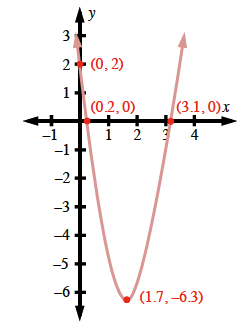### Home > CCA > Chapter 11 > Lesson 11.3.4 > Problem11-120

11-120.

Graph the parabola described by the following equation by finding its $x$-intercepts and vertex.

$y=3x^2−10x+2$

First, convert the equation to graphing form.

$\frac{\textit{y}}{3}=\textit{x}^2-\frac{10\textit{x}}{3}+\frac{2}{3}$

$\frac{\textit{y}}{3}+\frac{19}{9} =\textit{x}^2-\frac{10}{3}\textit{x}+\frac{25}{9}$

$\textit{y}=3\left(\textit{x}-\frac{5}{3}\right)^2-\frac{19}{3}$

The equation helps you find the coordinates of the vertex.

$\left(\frac{5}{3},\;-\frac{19}{3}\right)$

Substitute $0$ for $y$ to find the $x$-intercepts.
Substitute $0$ for $x$ to find the $y$-intercept.

$\left(\frac{5+\sqrt{19}}{3}\approx 3.1,\;0\right)\left(\frac{5-\sqrt{19}}{3}\approx 0.2,\;0\right) (0, 2)$